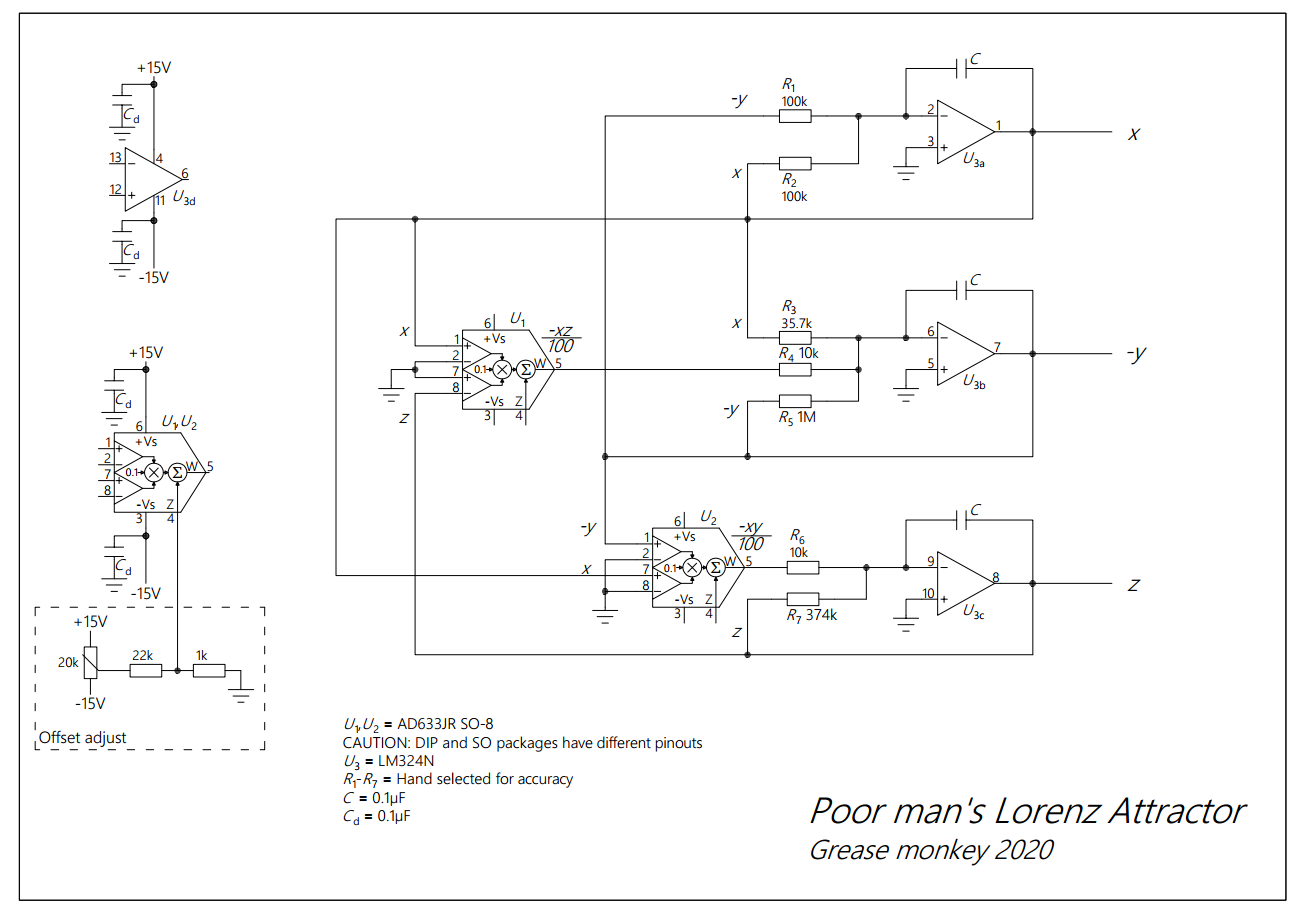May 28, 2020 Pointless, Chaos, Analog computing

# Poor man's Lorenz Attractor

I am making a circuit that solves the Lorenz Attractor differential equationsThe first time I came across the Lorenz attractor was in the book Chaos: Making a New Science. That was in 1996 (I was in high school back then). It is a system of three simple differential equations that exhibit a very complex behavior.

The Lorenz system: $${dx \over dt} = \sigma (y-x)$$ $${dy \over dt} = \rho x-y-xz$$ $${dz \over dt} = xy - \beta z$$ Parameter values: $\sigma=10$, $\beta={8 \over 3}$, $\rho=28$.

I recently saw a circuit that solves Lorenz's differential equations in The Art of Electronics: The x Chapters. So I thought "great, let's do it". But then I found out how much the MPY634 multiplier costs. Almost \$24 in Digikey. And you need two of them.

So what are the alternatives? The cheapest multiplier in stock was AD633. The difference between the two is mainly their precision (0.5% vs 2%). Guess what costs what. Would it work with ±2% error? (Spoiler: it does). And why not go dirt cheap with an LM324 op amp? This is the circuit I came up with:Schematic (enlarge, pdf)The breadboard circuit has a couple of components more than the schematic. I omitted them for the sake of simplicity. The 10-turn pot in series with a $330k$ resistor makes $R_7$ as close to $374k$ as possible. The jumpers short-circuit the inputs of $U_1$ and $U_2$ to ground in order trim their offset.

If you need the circuit to run at a different speed change the values of C. Smaller capacitors make it run faster. After some tries I found out that for my humble DS1052E 0.1μF is fine.

### Update 16.06.2020

I just got a new oscilloscope. So here's a video of the circuit's output.